﻿ AGV调度算法概述 - Nemo

# AGV调度算法概述Notice### 一 . AGV调度系统基本概念

• 调度系统（Dispatching system）：上位控制系统中用于任务调度、车辆管理及交通管理的控制软件，通常系统追求的是整体最优解。

• Dispatch（派遣）：指派一个AGV去执行一个运输任务。

• Schedule（狭义上的“调度”）：分配一批运输任务给一组AGV去执行。

• Route（路径规划）：生成所有被指定 AGV 的路径使其能完成各自被指派的任务。在 AGV 领域通常使用 route 表示，翻译为“路线、轨道”，它一般表示固定的不经常变动的路径。

• 实际AGV运行过程中遇到的冲突情况：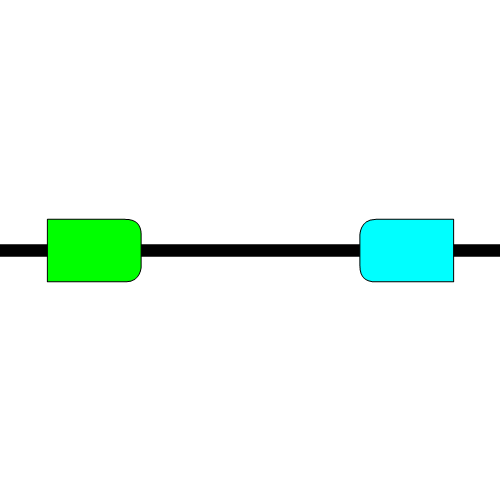当发生相向冲突时，会导致死锁现象的出现，造成道路拥堵问题；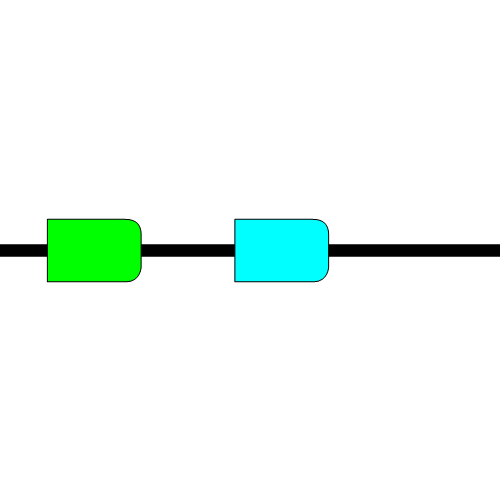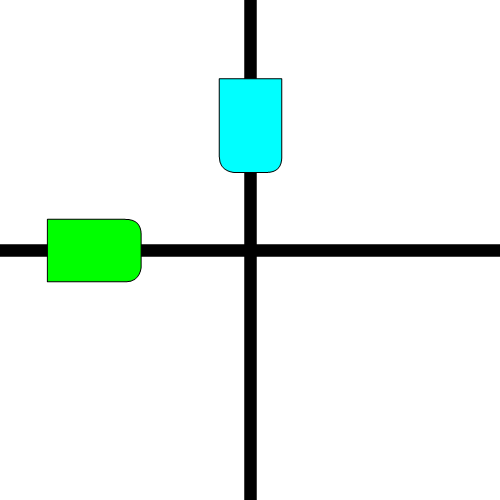### 二. 路径规划方法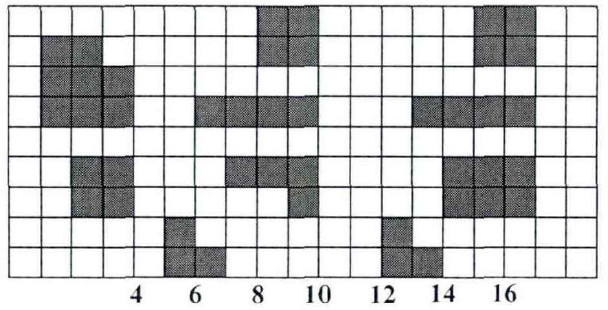AGV系统使用栅格法进行环境建模时，一般栅格的单位值为一台AGV宽度的一点几倍，以AGV刚好可以在并排的单元格无碰撞行走为宜，既可以满足定位精度的需要又可以节约存储空间、降低运算成本。

#### Dijkstra算法

Dijkstra算法的核心思想是以起点为中心向外逐步拓展搜寻，直至遍历到目标点为止：

• 首先将所有节点分成两部分，已遍历求得最短距离的节点集合Ｕ，以及未遍历节点集合Ｖ；
• 计算集合Ｖ中的节点与起点Ｓ的路程距离，按数值大小进行排序；
• 每个遍历周期选择距离值最小的节点添加进集合Ｕ，直至遍历目标点０出现在集合Ｕ。

#### A*算法

Ａ＊算法的核心思想是通过设置代价函数对未遍历点进行筛选，使搜索方向更偏向目标点，缩小搜索范围，进而提升搜索速度。路径成本表达式为：

f(n)=g(n)+h(n)

f(n)：起点Ｐ—遍历中间点ｎ—目标点ｇ的路径总成本；

g(n)：起点Ｐ—遍历中间点n之间的实际路径成本；

h(n)：遍历中间点—目标点０的预估路径成本。

• 新建两个列表用作路径数据点存储：openlist和closelist，openlist初始默认包含路径起点；
• 计算与起点相邻的节点的f(n)，按数值大小进行排序，f(n)最小节点m转移到closelist作为当前最优路径的过程点，其余节点留在openlist中做备用节点；
• 若m为目标点，则停止搜索，并输出最优路径结果，否则继续；
• 计算遍历中间栅格点ｍ的所有相邻栅格点的f(n)，若相邻节点n未加入openlist，及时添加，并令g(n)=g(m)+d(m,n)，此时ｎ的父节点为m；若相邻点n在openlist中，当g(n)>g(m)+d(m,n)，更新g(n)=g(m)+d(m,n)，设置n的父节点为m；
• 遍历完所有备选点后重复步骤（２）-（４），直到目标点Ｑ出现在openlist中，搜索结束，按进入closelist的先后顺序输出中的节点栅格序列，该序列即为最优路径。

#### 蚁群算法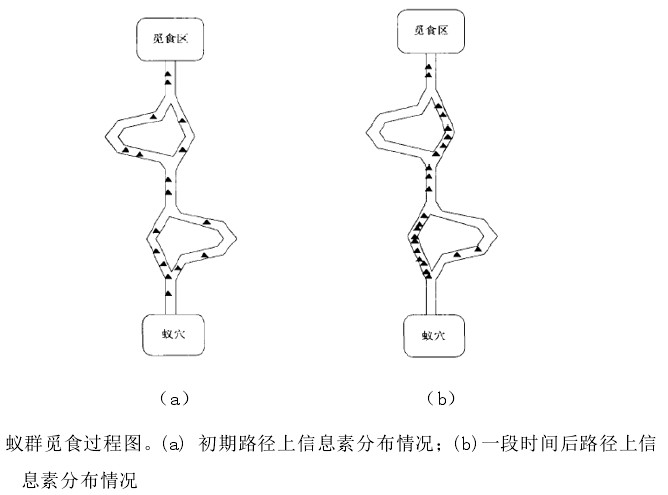1. 概率转移规则

在初始时刻，采用栅格法建模完成地图的构建，并初始化地图中各条路径上的信息素初始值。然后设置蚁群优化算法的参数，将m只蚂蚁全部放置于起点。蚂蚁k 在 t 时刻从栅格 i 到栅格 j 的转移概率为 P_{i j}^{k}(t)，其表达式为:

P_{i j}^{k}(t)=\left{\begin{array}{l}
\frac{\left[\tau_{i j}(t)\right]^{\alpha} \cdot\left[\eta_{i j}(t)\right]^{\beta}}{\sum_{s \in \text {allow}_{k}}\left[\tau_{i s}(t)\right]^{\alpha} \cdot\left[\eta_{i s}(t)\right]^{\beta}}, s \in \text {allow}_{k} \
0, s \notin \text {allow}_{k}
\end{array}\right.

其中：

• $n_{ij}(k)$ ——t 时刻蚂蚁从栅格 i 转移到栅格 j 的启发信息；

• $\tau_{ij}(t)$—— t 时刻栅格 i 到栅格 j 上残留的信息素浓度；

• $\alpha$——信息启发函数的重要程度因子，即蚂蚁在运动过程中路径上的信息素在蚂蚁选择路径中重要程度，其值越大则后面的蚂蚁在进行搜索时更倾向于其它蚂蚁行走过得路径；

• $\beta$——信息素的重要程度因子，即蚂蚁在运动过程中启发信息在蚂蚁选择路径中重要程度，其值越大蚂蚁选择最短路径行走，从而演变为贪心算法；

• $\text {allow}_{k}$——蚂蚁 k 下次允许访问栅格的集合，蚂蚁下次访问栅格为当前栅格 i 的上、下、左、右、左上、左下、右上、右下八个栅格，排除障碍物栅格、已走过栅格等禁忌栅格，即为允许访问栅格的集合；

• $\eta_{i j}(t)$——能见度，其计算公式为： $\eta_{i j}(t)=1 / d_{i j}$，其中$d_{i j}$为栅格 i 到栅格 j 的距离。

1. 信息素更新规则

信息素更新分为局部信息素更新全局信息素更新

全局信息素更新：即当所有蚂蚁完成一次循环后需要对各个栅格连接的路径信息素浓度进行更新，其公式为：

\eta_{i j}(t)=1 / d_{i j}\left{\begin{array}{l}
\tau_{i j}(t+1)=(1-\rho) \tau_{i j}(t)+\Delta \tau_{i j} \
\Delta \tau_{i j}=\sum_{k=1}^{m} \Delta \tau_{i j}^{k}
\end{array}, 0<\rho<1\right.

其中：

• $m$——蚁群中蚂蚁数目;

• $\rho$——信息素的挥发系数；

• $\Delta \tau_{i j} ^k$——第 k 只蚂蚁在栅格 i 到栅格 j 路径上释放的信息素浓度，其信息素浓度视蚂蚁经过此条路径完成路径搜索总长度而定，路径越短则信息素浓度越高；

• $\Delta \tau_{i j}$——所有蚂蚁在栅格 i到栅格 j 路径上释放的信息素浓度总和。

蚂蚁释放信息素采用的是 ant_cycle_system 模型，其主要是利用蚂蚁经过路径的整体信息来计算释放信息素浓度：

\Delta \tau_{i j}^{k}=\left{\begin{array}{ll}Q / L_{k},&\text { 当蚂蚁 } k \text { 经过路径 }(i, j) \text { 时 }\\0,&\text { 当蚂蚁 } k \text { 不经过路径 }(i, j) \text { 时 }\end{array}\right.

其中：

• $Q$——信息素强度，即蚂蚁循环一次释放的信息素总量；
• $L_{k}$——第 K 只蚂蚁在本次循环中从起点到目标点进行路径搜索所经过路径的长度。
1. 优化方向
1. 蚂蚁数目 ：蚁群数目增加，算法中每次迭代的计算量也会增加。蚁群数目过小，全局搜索随机性越弱，容易导致算法出现过早停滞现象。
2. 重要程度因子α ：重要程度因子过小，信息素的重要性不能充分体现，导致算法收敛速度过慢且易于陷入局部最优。而重要程度因子过大，信息素的重要性会得以充分体现，局部最优路径上的正反馈作用会过强，导致算法过早收敛。
3. 期望启发式因子β ：期望启发式因子过小，蚁群算法会陷入纯粹的随机搜索，导致算法很难找到最优解。而期望启发式因子过大，路径搜索时选择局部最短路径的可能性越大，算法收敛速度虽然增快，但是全局搜索能力变差导致陷入局部最优。
4. 信息素挥发因子ρ ：信息素挥发因子过大，则路径上留下的信息素挥发过快以至于减小到零，会导致算法重复搜索可能性过大，影响算法的随机性和全局搜索能力。而信息素挥发因子过小，则会导致算法收敛速度降低。
5. 信息素强度 ：信息素强度越大，则算法已搜索过路径信息素积累越快，导致算法收敛速度越快，但是使得搜索空间减小而陷入局部最优的可能性增大。而信息素强度越小， 作用相反。

### 三. AGV调度方法

#### 1. AGV 系统调度原则

1. 行驶路径最短原则

在对任务进行调度时，遵循总的行驶路径最短的原则对任务进行路径分配和优化，采用此原则虽然能够保证 AGV 行驶路径最短，但是可能会延长任务的行驶时间和行驶成本。

2. 等待时间最短原则

是指在进行任务调度时使总的等待时间最短，这里的等待时间主要包括空闲等待时间和冲突等待时间。空闲等待时间是指在地面控制系统收到工位的请求任务时，系统在响应该任务所需的等待时间；冲突等待时间是针对于多 AGV 系统中，AGV 在运行过程中出现路径冲突时所需等待的时间。

3. AGV 配置数量最少原则

综合各方面的因素，在保证工厂物流运输效率的前提下，AGV 的数量因尽可能减少，这样不仅可以减少成本，而且可以提高 AGV 的利用率。

4. 成本最少原则

系统的成本是由系统资源决定的，减少成本实质就是将有限的系统资源发挥最大的功能。

### 引用Nemo版权所有丨如未注明，均为原创丨本网站采用BY-NC-SA协议进行授权，转载请注明转自：https://nemo.cool/652.html
# # #### 推荐文章AGV调度算法概述
 Notice动图来源：CSDN:robinvista 版权为原作所有 一 . AGV调度系统基本概念 调度系统（Dispatching system）：上位控制系统中用于任务调度、车辆管理及交通管理的控制软件…

2020-08-12